# RS Aggarwal Solutions for Class 8 Maths Chapter 13 - Time and Work

RS Aggarwal Solutions for Class 8 Maths Chapter 13- Time and Work, are provided here. Our expert faculty team has prepared solutions in order to help you with your exam preparation to acquire good marks in Maths. If you wish to secure an excellent score, solving RS Aggarwal Solutions for Class 8 is an utmost necessity. These solutions will help you in gaining knowledge and strong command over the subject. Practising the textbook questions will help you in analyzing your level of preparation and knowledge of the concept.

In order to help you understand and solve the problems, we at BYJU’S have designed these solutions in a very lucid and clear manner that helps students solve problems in the most efficient possible ways. Here is the RS Aggarwal Solutions for Class 8 Chapter 13 where solutions are solved in detail. Download pdf of Class 8 Chapter 13 in their respective links.

## Download PDF of RS Aggarwal Solutions for Class 8 Maths Chapter 13 – Time and Work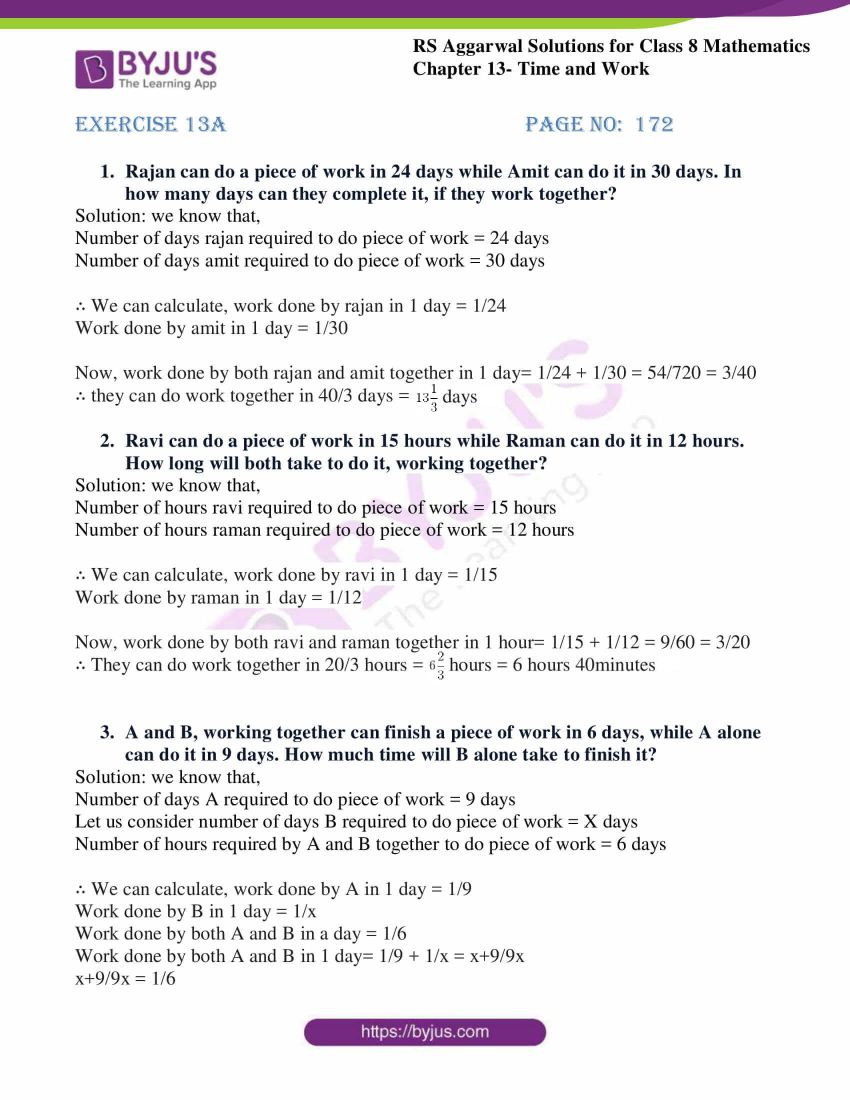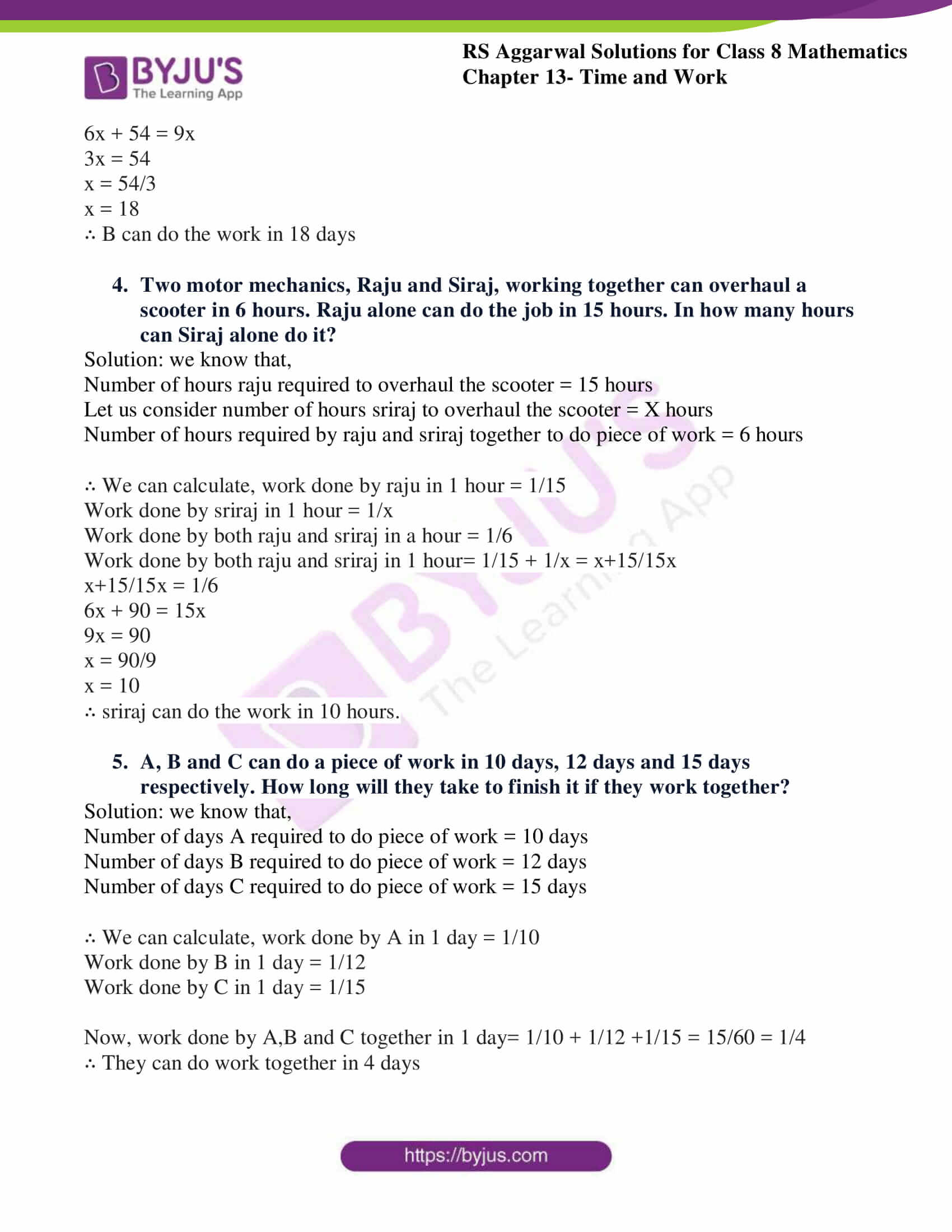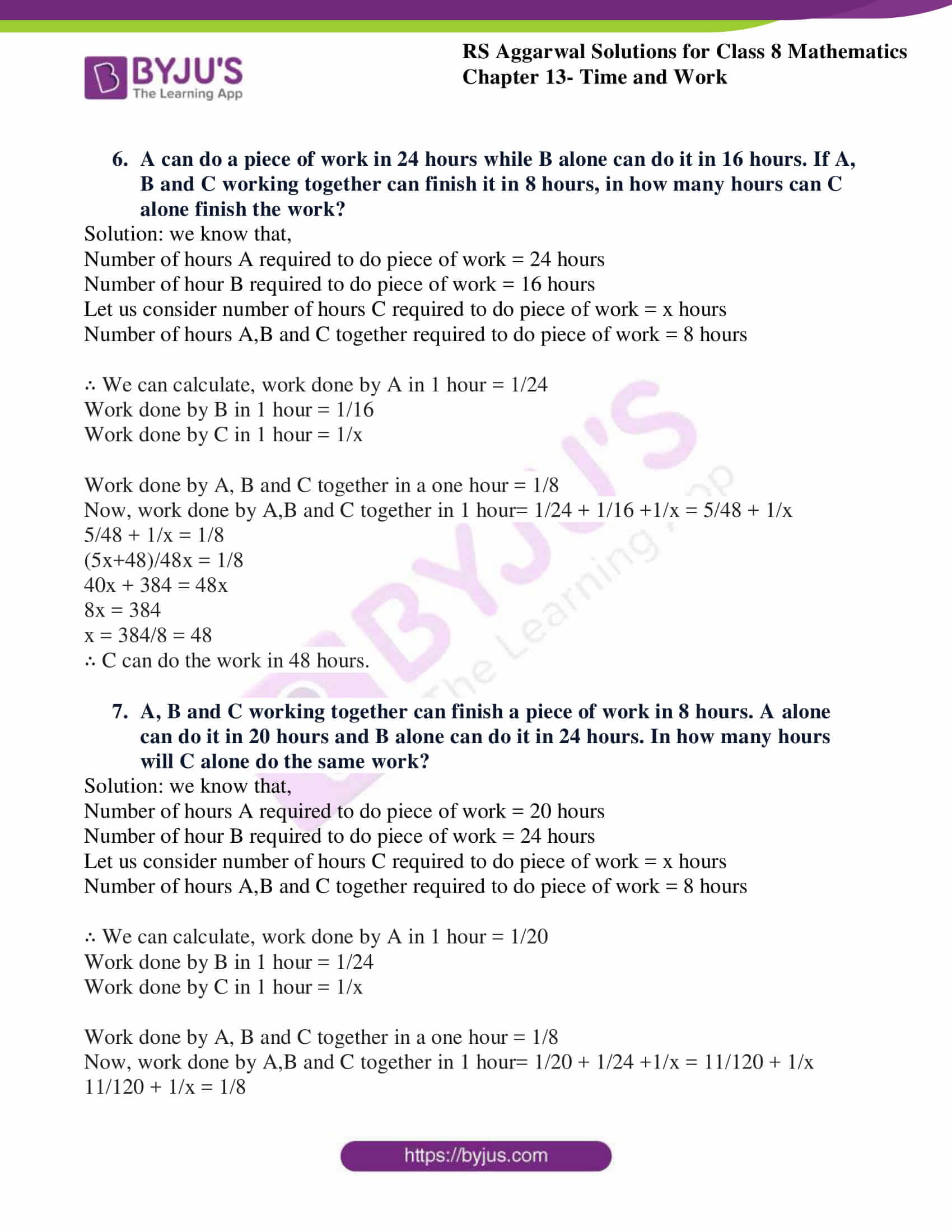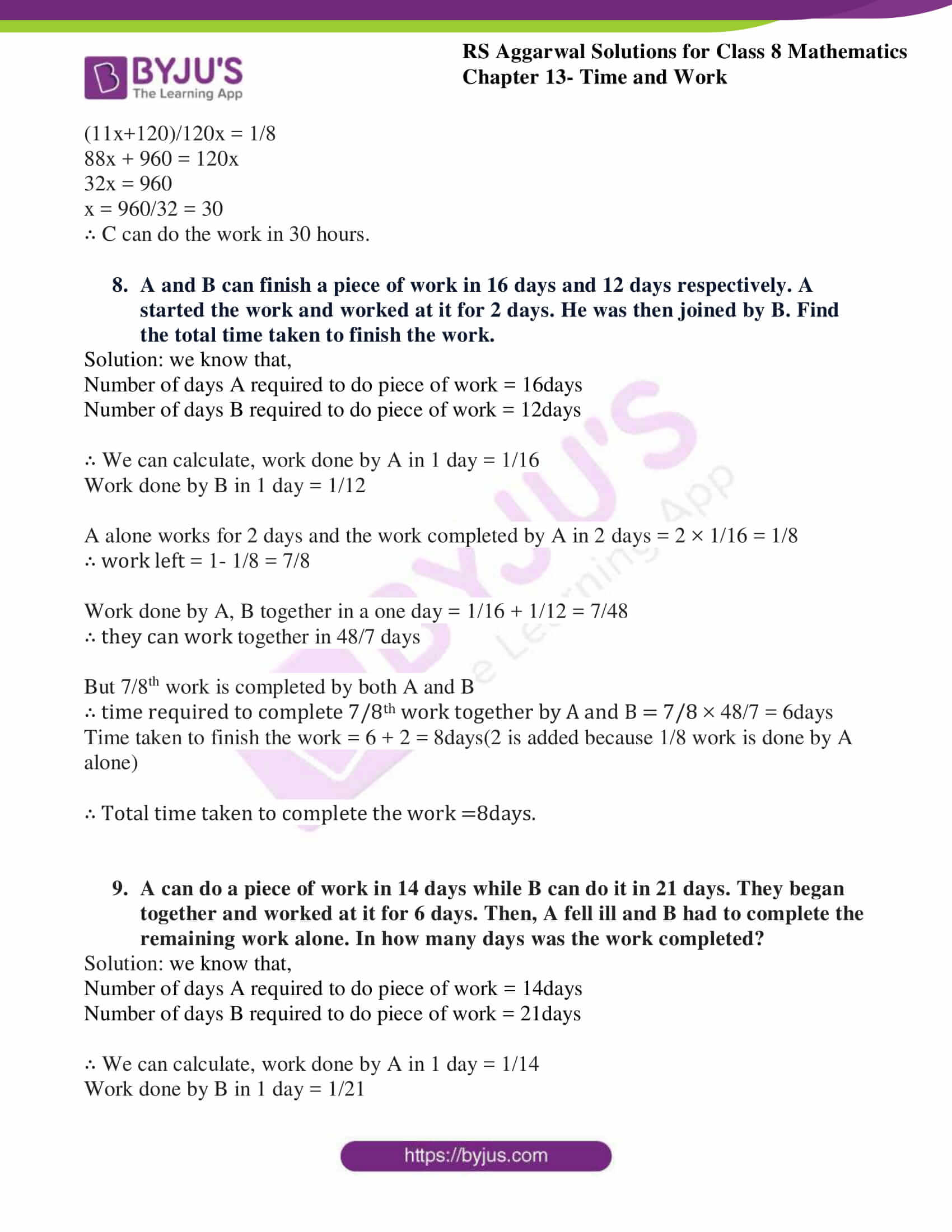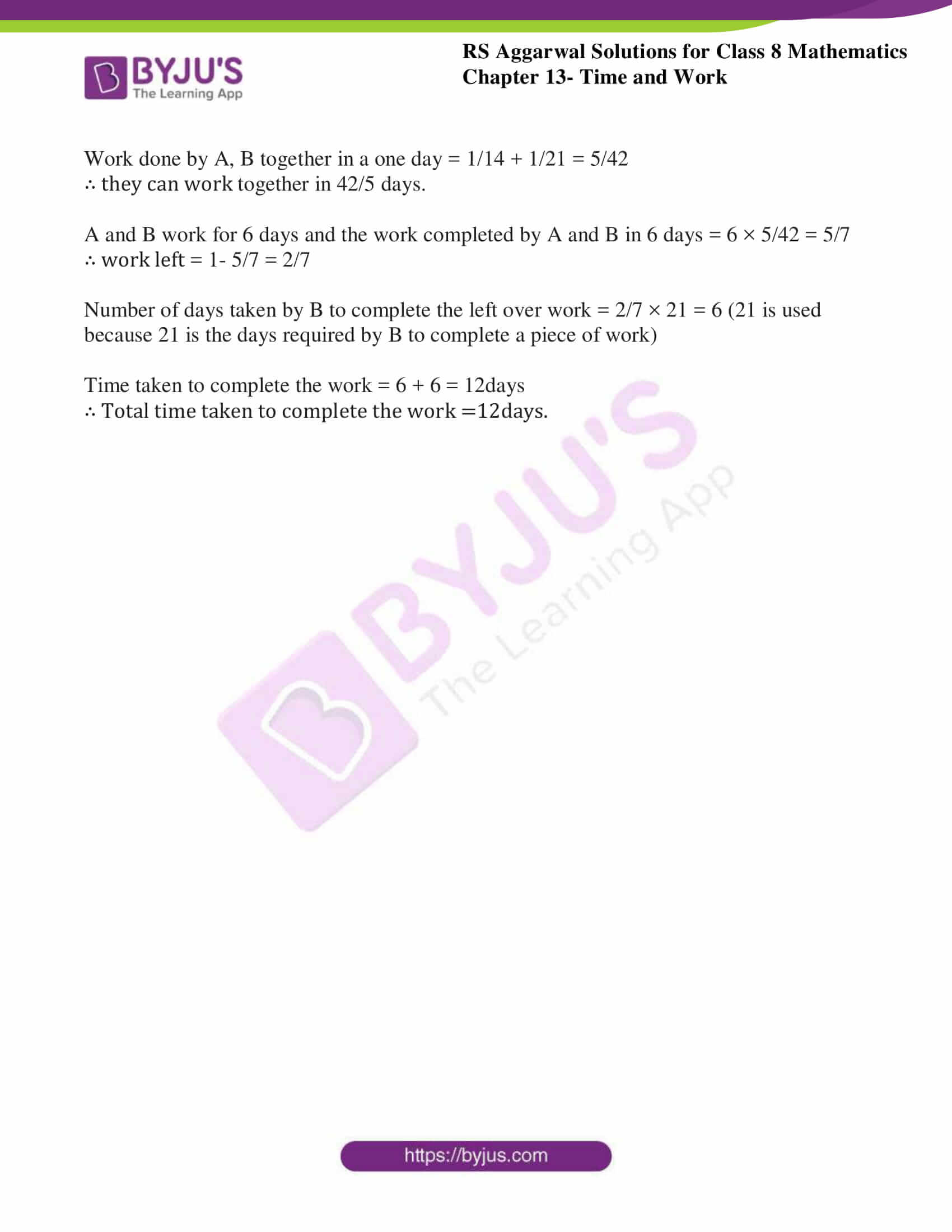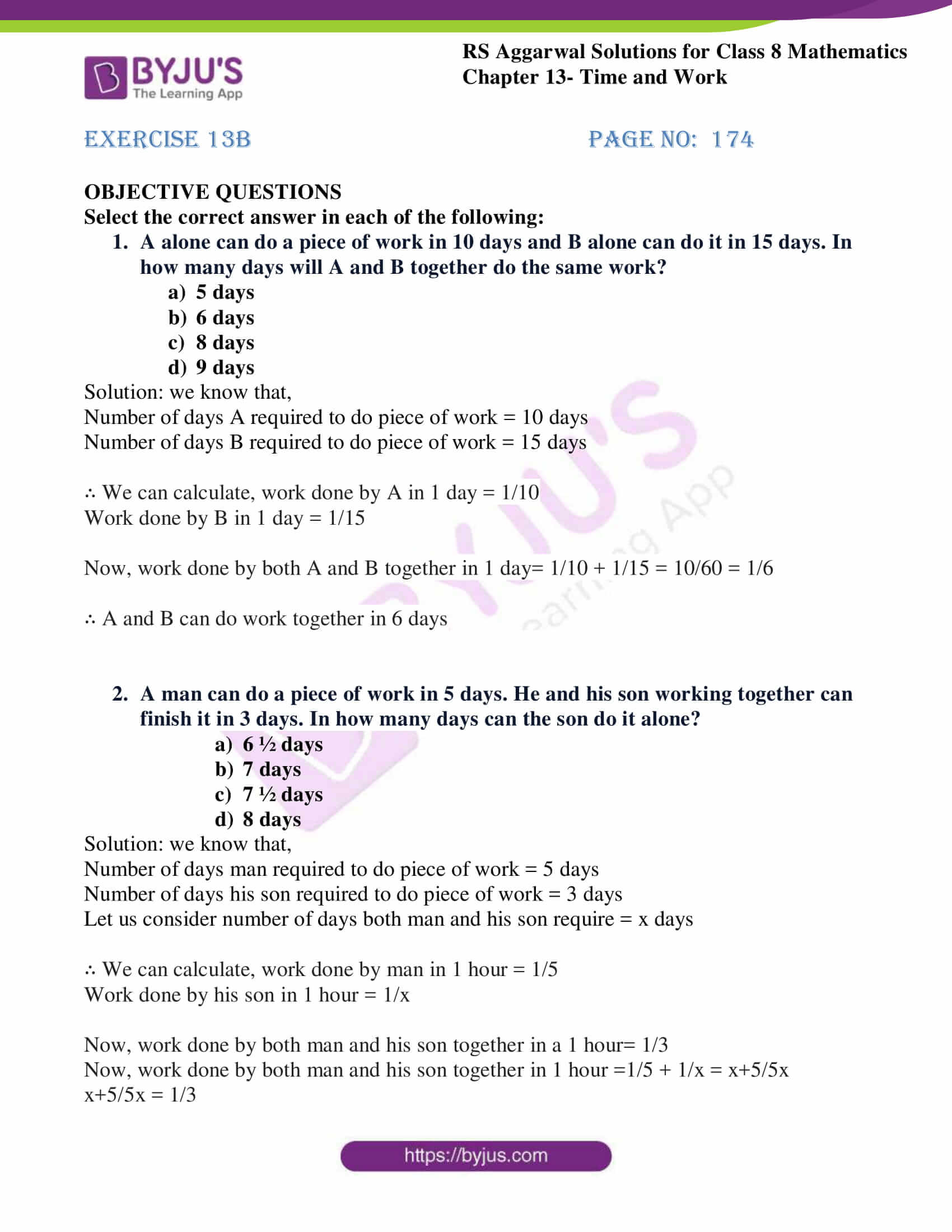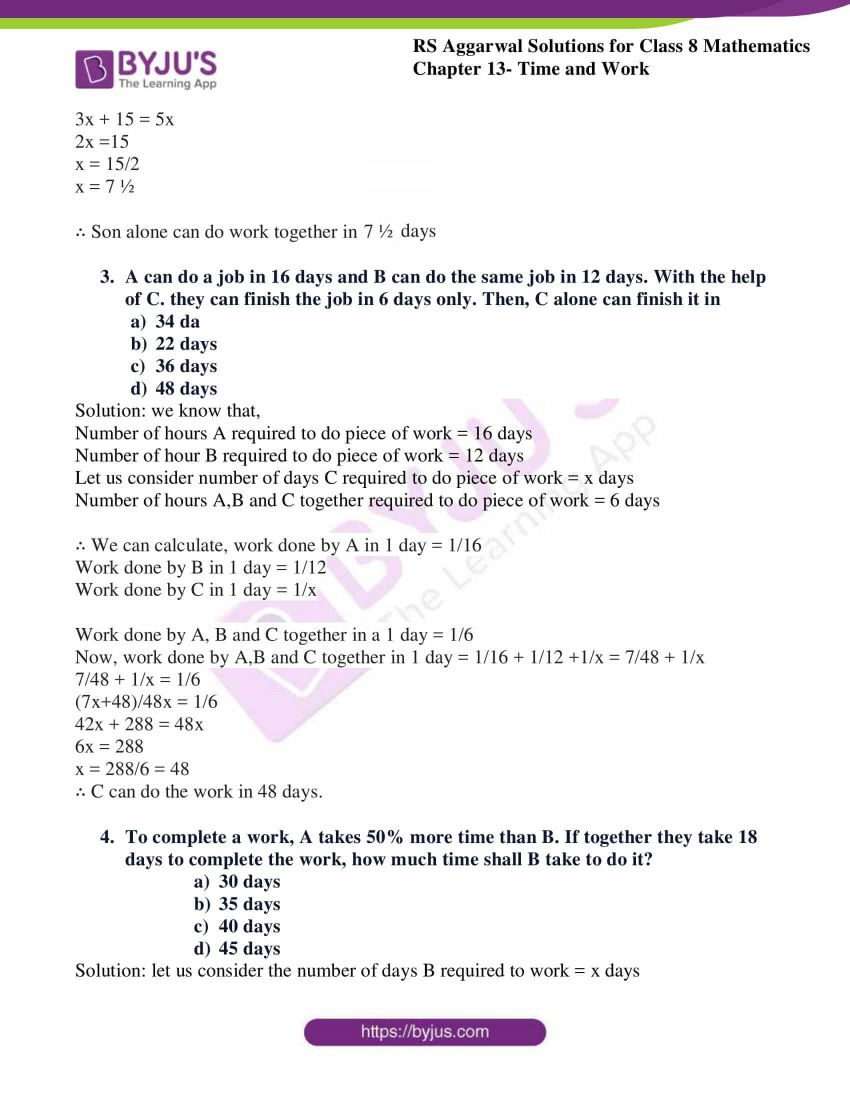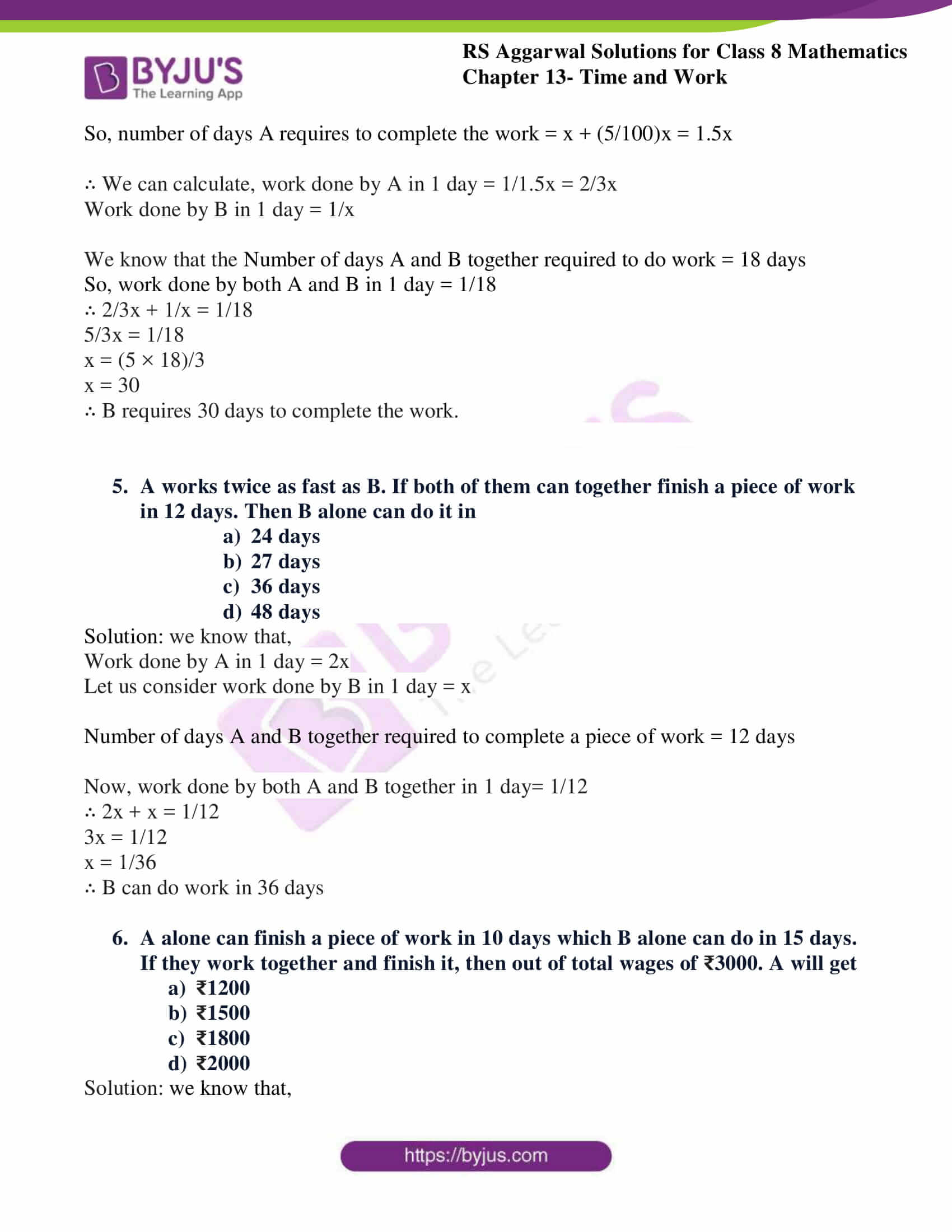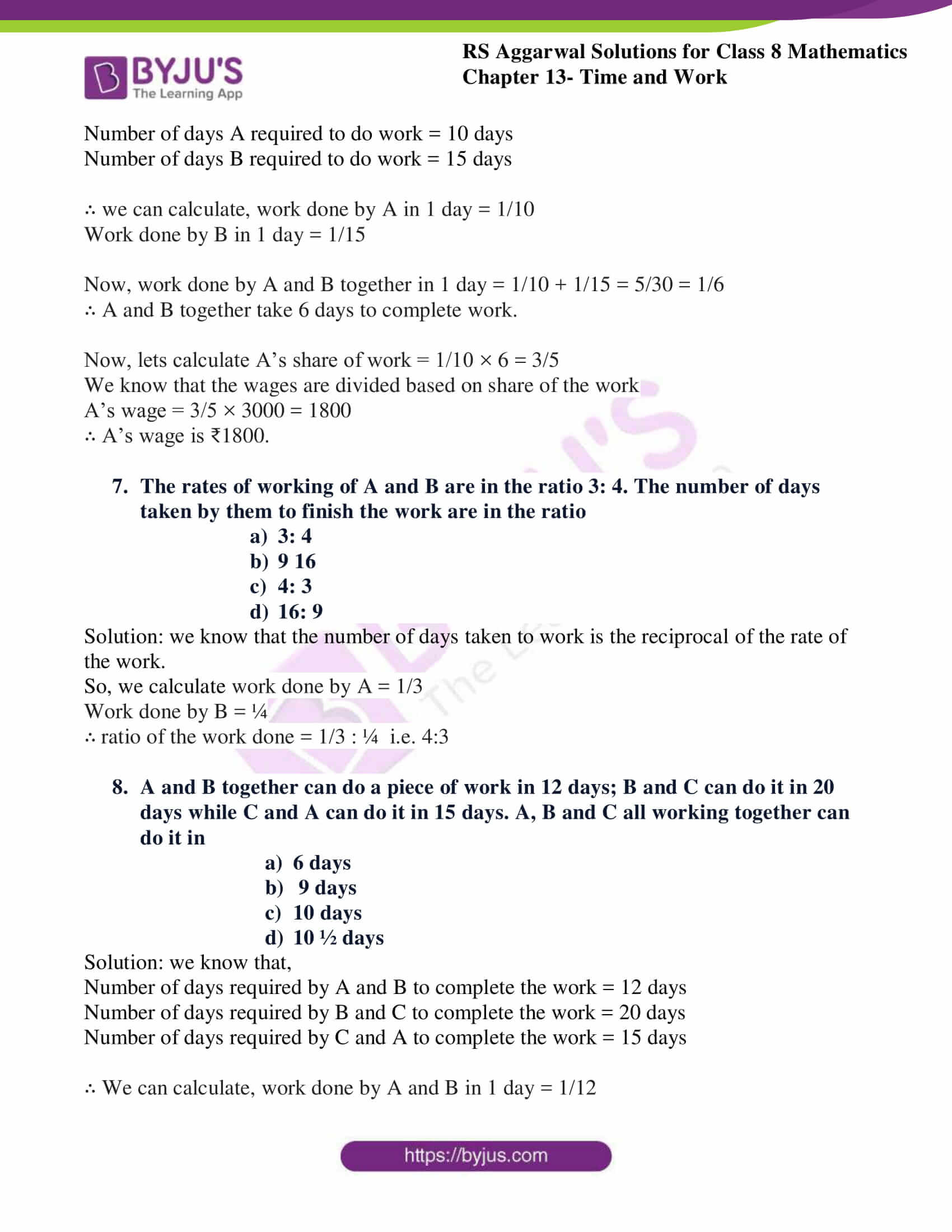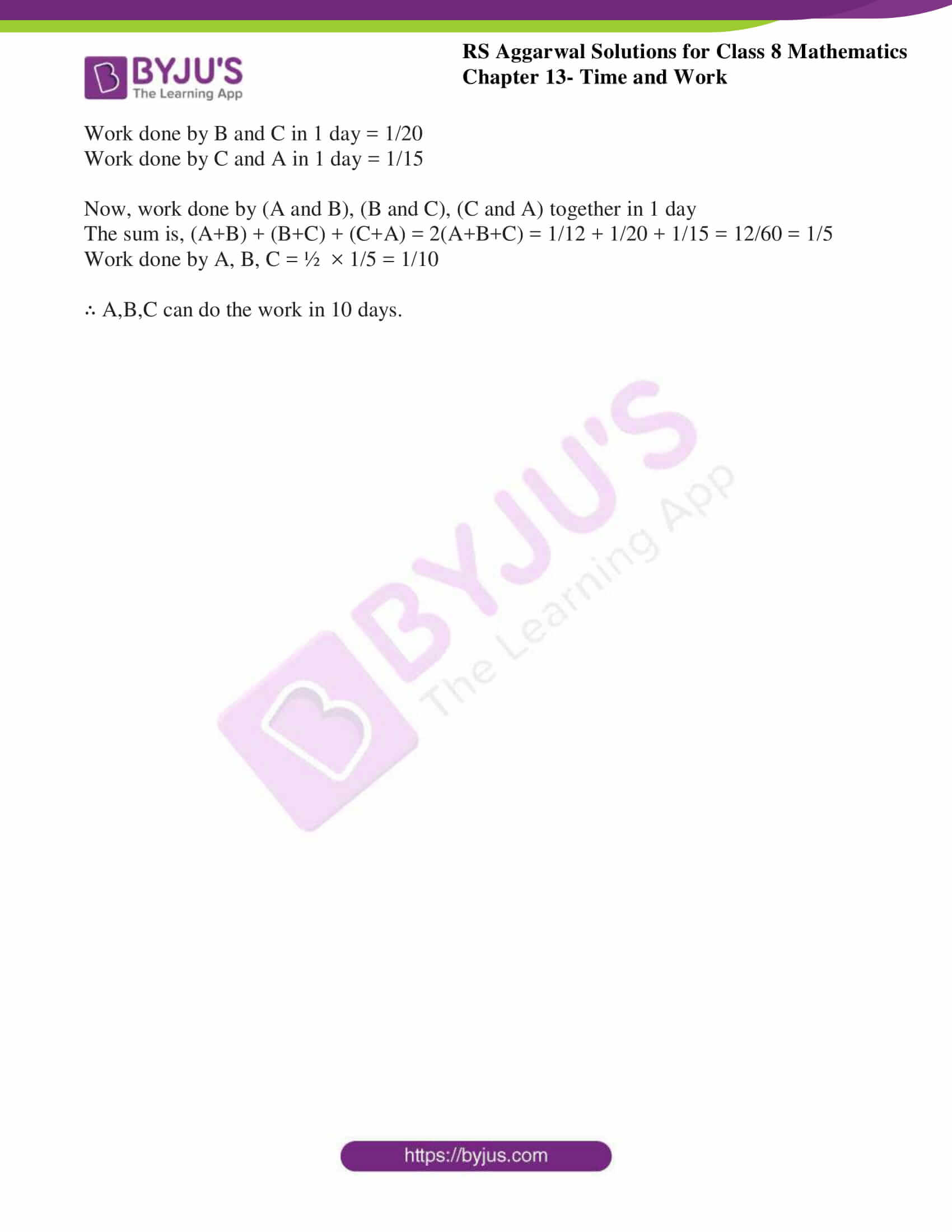## Exercise 13A

1. Rajan can do a piece of work in 24 days while Amit can do it in 30 days. In how many days can they complete it, if they work together?

Solution: we know that,

Number of days Rajan required to do piece of work = 24 days

Number of days Amit required to do piece of work = 30 days

∴ We can calculate, work done by Rajan in 1 day = 1/24

Work done by Amit in 1 day = 1/30

Now, work done by both Rajan and Amit together in 1 day= 1/24 + 1/30 = 54/720 = 3/40

∴ they can do work together in 40/3 days = $13\frac{1}{3}$ days

2. Ravi can do a piece of work in 15 hours while Raman can do it in 12 hours. How long will both take to do it, working together?

Solution: we know that,

Number of hours Ravi required to do piece of work = 15 hours

Number of hours Raman required to do piece of work = 12 hours

∴ We can calculate, work done by Ravi in 1 day = 1/15

Work done by Raman in 1 day = 1/12

Now, work done by both Ravi and Raman together in 1 hour= 1/15 + 1/12 = 9/60 = 3/20

∴ They can do work together in 20/3 hours = $6\frac{2}{3}$ hours = 6 hours 40minutes

3. A and B, working together can finish a piece of work in 6 days, while A alone can do it in 9 days. How much time will B alone take to finish it?

Solution: we know that,

Number of days A required to do piece of work = 9 days

Let us consider number of days B required to do piece of work = X days

Number of hours required by A and B together to do piece of work = 6 days

∴ We can calculate, work done by A in 1 day = 1/9

Work done by B in 1 day = 1/x

Work done by both A and B in a day = 1/6

Work done by both A and B in 1 day= 1/9 + 1/x = x+9/9x

x+9/9x = 1/6

6x + 54 = 9x

3x = 54

x = 54/3

x = 18

∴ B can do the work in 18 days

4. Two motor mechanics, Raju and Siraj, working together can overhaul a scooter in 6 hours. Raju alone can do the job in 15 hours. In how many hours can Siraj alone do it?

Solution: we know that,

Number of hours Raju required to overhaul the scooter = 15 hours

Let us consider number of hours Sriraj to overhaul the scooter = X hours

Number of hours required by Raju and Sriraj together to do piece of work = 6 hours

∴ We can calculate, work done by Raju in 1 hour = 1/15

Work done by Sriraj in 1 hour = 1/x

Work done by both Raju and Sriraj in a hour = 1/6

Work done by both Raju and Sriraj in 1 hour= 1/15 + 1/x = x+15/15x

x+15/15x = 1/6

6x + 90 = 15x

9x = 90

x = 90/9

x = 10

∴ Sriraj can do the work in 10 hours.

5. A, B and C can do a piece of work in 10 days, 12 days and 15 days respectively. How long will they take to finish it if they work together?

Solution: we know that,

Number of days A required to do piece of work = 10 days

Number of days B required to do piece of work = 12 days

Number of days C required to do piece of work = 15 days

∴ We can calculate, work done by A in 1 day = 1/10

Work done by B in 1 day = 1/12

Work done by C in 1 day = 1/15

Now, work done by A,B and C together in 1 day= 1/10 + 1/12 +1/15 = 15/60 = 1/4

∴ They can do work together in 4 days

6. A can do a piece of work in 24 hours while B alone can do it in 16 hours. If A, B and C working together can finish it in 8 hours, in how many hours can C alone finish the work?

Solution: we know that,

Number of hours A required to do piece of work = 24 hours

Number of hour B required to do piece of work = 16 hours

Let us consider number of hours C required to do piece of work = x hours

Number of hours A,B and C together required to do piece of work = 8 hours

∴ We can calculate, work done by A in 1 hour = 1/24

Work done by B in 1 hour = 1/16

Work done by C in 1 hour = 1/x

Work done by A, B and C together in a one hour = 1/8

Now, work done by A,B and C together in 1 hour= 1/24 + 1/16 +1/x = 5/48 + 1/x

5/48 + 1/x = 1/8

(5x+48)/48x = 1/8

40x + 384 = 48x

8x = 384

x = 384/8 = 48

∴ C can do the work in 48 hours.

7. A, B and C working together can finish a piece of work in 8 hours. A alone can do it in 20 hours and B alone can do it in 24 hours. In how many hours will C alone do the same work?

Solution: we know that,

Number of hours A required to do piece of work = 20 hours

Number of hour B required to do piece of work = 24 hours

Let us consider number of hours C required to do piece of work = x hours

Number of hours A,B and C together required to do piece of work = 8 hours

∴ We can calculate, work done by A in 1 hour = 1/20

Work done by B in 1 hour = 1/24

Work done by C in 1 hour = 1/x

Work done by A, B and C together in a one hour = 1/8

Now, work done by A,B and C together in 1 hour= 1/20 + 1/24 +1/x = 11/120 + 1/x

11/120 + 1/x = 1/8

(11x+120)/120x = 1/8

88x + 960 = 120x

32x = 960

x = 960/32 = 30

∴ C can do the work in 30 hours.

8. A and B can finish a piece of work in 16 days and 12 days respectively. A started the work and worked at it for 2 days. He was then joined by B. Find the total time taken to finish the work.

Solution: we know that,

Number of days A required to do piece of work = 16 days

Number of days B required to do piece of work = 12 days

∴ We can calculate, work done by A in 1 day = 1/16

Work done by B in 1 day = 1/12

A alone works for 2 days and the work completed by A in 2 days = 2 × 1/16 = 1/8

∴ work left = 1- 1/8 = 7/8

Work done by A, B together in a one day = 1/16 + 1/12 = 7/48

∴ they can work together in 48/7 days

But 7/8th work is completed by both A and B

∴ time required to complete 7/8th work together by A and B = 7/8 × 48/7 = 6days

Time taken to finish the work = 6 + 2 = 8days(2 is added because 1/8 work is done by A alone)

∴ Total time taken to complete the work =8days.

9. A can do a piece of work in 14 days while B can do it in 21 days. They began together and worked at it for 6 days. Then, A fell ill and B had to complete the remaining work alone. In how many days was the work completed?

Solution: we know that,

Number of days A required to do piece of work = 14 days

Number of days B required to do piece of work = 21 days

∴ We can calculate, work done by A in 1 day = 1/14

Work done by B in 1 day = 1/21

Work done by A, B together in a one day = 1/14 + 1/21 = 5/42

∴ they can work together in 42/5 days.

A and B work for 6 days and the work completed by A and B in 6 days = 6 × 5/42 = 5/7

∴ work left = 1- 5/7 = 2/7

Number of days taken by B to complete the left over work = 2/7 × 21 = 6 (21 is used because 21 is the days required by B to complete a piece of work)

Time taken to complete the work = 6 + 6 = 12days

∴ Total time taken to complete the work =12days.

## Exercise 13B

Select the correct answer in each of the following:

1. A alone can do a piece of work in 10 days and B alone can do it in 15 days. In how many days will A and B together do the same work?

1. 5 days
2. 6 days
3. 8 days
4. 9 days

Solution: we know that,

Number of days A required to do piece of work = 10 days

Number of days B required to do piece of work = 15 days

∴ We can calculate, work done by A in 1 day = 1/10

Work done by B in 1 day = 1/15

Now, work done by both A and B together in 1 day= 1/10 + 1/15 = 10/60 = 1/6

∴ A and B can do work together in 6 days

2. A man can do a piece of work in 5 days. He and his son working together can finish it in 3 days. In how many days can the son do it alone?

1. 6 ½ days
2. 7 days
3. 7 ½ days
4. 8 days

Solution: we know that,

Number of days man required to do piece of work = 5 days

Number of days his son required to do piece of work = 3 days

Let us consider number of days both man and his son require = x days

∴ We can calculate, work done by man in 1 hour = 1/5

Work done by his son in 1 hour = 1/x

Now, work done by both man and his son together in a 1 hour= 1/3

Now, work done by both man and his son together in 1 hour =1/5 + 1/x = x+5/5x

x+5/5x = 1/3

3x + 15 = 5x

2x =15

x = 15/2

x = 7 ½

∴ Son alone can do work together in 7 ½ days

3. A can do a job in 16 days and B can do the same job in 12 days. With the help of C. they can finish the job in 6 days only. Then, C alone can finish it in

1. 34 days
2. 22 days
3. 36 days
4. 48 days

Solution: we know that,

Number of hours A required to do piece of work = 16 days

Number of hour B required to do piece of work = 12 days

Let us consider number of days C required to do piece of work = x days

Number of hours A,B and C together required to do piece of work = 6 days

∴ We can calculate, work done by A in 1 day = 1/16

Work done by B in 1 day = 1/12

Work done by C in 1 day = 1/x

Work done by A, B and C together in a 1 day = 1/6

Now, work done by A,B and C together in 1 day = 1/16 + 1/12 +1/x = 7/48 + 1/x

7/48 + 1/x = 1/6

(7x+48)/48x = 1/6

42x + 288 = 48x

6x = 288

x = 288/6 = 48

∴ C can do the work in 48 days.

4. To complete a work, A takes 50% more time than B. If together they take 18 days to complete the work, how much time shall B take to do it?

1. 30 days
2. 35 days
3. 40 days
4. 45 days

Solution: let us consider the number of days B required to work = x days

So, number of days A requires to complete the work = x + (5/100)x = 1.5x

∴ We can calculate, work done by A in 1 day = 1/1.5x = 2/3x

Work done by B in 1 day = 1/x

We know that the Number of days A and B together required to do work = 18 days

So, work done by both A and B in 1 day = 1/18

∴ 2/3x + 1/x = 1/18

5/3x = 1/18

x = (5 × 18)/3

x = 30

∴ B requires 30 days to complete the work.

5. A works twice as fast as B. If both of them can together finish a piece of work in 12 days. Then B alone can do it in

1. 24 days
2. 27 days
3. 36 days
4. 48 days

Solution: we know that,

Work done by A in 1 day = 2x

Let us consider work done by B in 1 day = x

Number of days A and B together required to complete a piece of work = 12 days

Now, work done by both A and B together in 1 day= 1/12

∴ 2x + x = 1/12

3x = 1/12

x = 1/36

∴ B can do work in 36 days

6. A alone can finish a piece of work in 10 days which B alone can do in 15 days. If they work together and finish it, then out of total wages of ₹3000. A will get

1. ₹1200
2. ₹1500
3. ₹1800
4. ₹2000

Solution: we know that,

Number of days A required to do work = 10 days

Number of days B required to do work = 15 days

∴ we can calculate, work done by A in 1 day = 1/10

Work done by B in 1 day = 1/15

Now, work done by A and B together in 1 day = 1/10 + 1/15 = 5/30 = 1/6

∴ A and B together take 6 days to complete work.

Now, lets calculate A’s share of work = 1/10 × 6 = 3/5

We know that the wages are divided based on share of the work

A’s wage = 3/5 × 3000 = 1800

∴ A’s wage is ₹1800.

7. The rates of working of A and B are in the ratio 3: 4. The number of days taken by them to finish the work are in the ratio

1. 3:4
2. 9:16
3. 4:3
4. 16: 9

Solution: we know that the number of days taken to work is the reciprocal of the rate of the work.

So, we calculate work done by A = 1/3

Work done by B = ¼

∴ ratio of the work done = 1/3 : ¼ i.e. 4:3

8. A and B together can do a piece of work in 12 days; B and C can do it in 20 days while C and A can do it in 15 days. A, B and C all working together can do it in

1. 6 days
2. 9 days
3. 10 days
4. 10 ½ days

Solution: we know that,

Number of days required by A and B to complete the work = 12 days

Number of days required by B and C to complete the work = 20 days

Number of days required by C and A to complete the work = 15 days

∴ We can calculate, work done by A and B in 1 day = 1/12

Work done by B and C in 1 day = 1/20

Work done by C and A in 1 day = 1/15

Now, work done by (A and B), (B and C), (C and A) together in 1 day

The sum is, (A+B) + (B+C) + (C+A) = 2(A+B+C) = 1/12 + 1/20 + 1/15 = 12/60 = 1/5

Work done by A, B, C = ½ × 1/5 = 1/10

∴ A,B,C can do the work in 10 days.

## RS Aggarwal Solutions for Class 8 Maths Chapter 13 – Time and Work

Chapter 13- Time and Work contains 2 exercises and the RS Aggarwal solutions present on this page provide the solutions for the questions present in each exercise. Now, let us have a look at the concepts discussed in this chapter.

• Introduction to Time and Work.
• Problems on pipes and cistern.

Exercise 13A

Exercise 13B

### Chapter Brief of RS Aggarwal Solutions for Class 8 Maths Chapter 13 – Time and Work

RS Aggarwal Solutions for Class 8 Maths Chapter 13 – Time and Work, ensures that the students are thorough and familiar with the concepts. Regular revision of important concepts and formulas over time to time is the best way to strengthen your concepts.

As the Chapter is about Time and Work. Here are some instances where we come across, we use them in calculating the distance, speed and time. For example, ‘x’ men required to complete a piece of work at a specified time. Here in the RS Aggarwal Solutions book, many such exercise problems are given which enhances familiarity with the concepts.